HistoryMathematiciansAll formulasNum. approx.SoftwaresMisc. math.DigitsPoetryPapers/videosDelirium !Pi-DayImagesMusicLinksBibliography Boris Gourévitch The world of Pi - V2.57 modif. 13/04/2013HomeVersion historyGuestbookWho I amSome pictures (fr)AcknowledgementsLast modificationsContact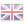### 2 Introduction to hypergeometric series

#### 2.1 Definition

An general hypergeometric function is defined by the serie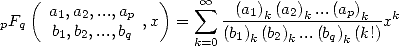(1)

where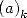is Pochhammer's symbol and is equal to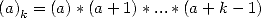It's a generalisation of the factorial function. A simple example of the value of Pochhammer's symbol is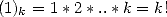An very important example of the value of Pochhmmer's symbol concern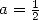: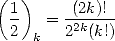(2)

In fact,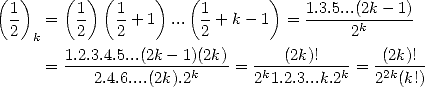which is equivalent to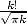when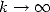due to Stirling's equivalence, so you have an idea.

In truth, we get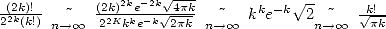.

In fact, there is a simple way to check if a serie can be put under a hypergeometric serie form. For this we calculate the ratio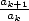and we try to put it under the form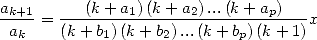(4)

that is a polynomial ratio in. Under this form, we can then precise that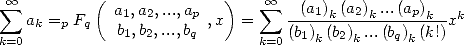(5)

Generally, for the series that don't give any simple results, we can often go back to study an integral involving the logarithm to the numerator (eventually at a certain power) and of the polynomials (eventually at a certain non integer power) i.e.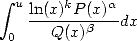(6)

#### 2.2 A few properties and simple examples

The ratio between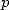andis very important so to known toward which kind of result we are going to. Because the result of a hypergeometric series with a fixed difference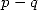are ”homogenous”, that is the closed form are always combinations of the same kind of basic function. (Of course, the combination can be very complicated, but it is important that we always see the same basic function appearing)

For example, the simple example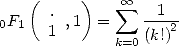(7)

leads the result of the series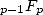toward Bessel's function :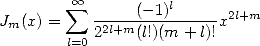(8)

for Bessel's function of first sort for example (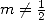), while the other serie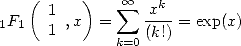(9)

indicates the variation of the coefficients for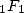(and more generally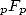) give us functions based on the exponentianal function. This, as we have often seen, does not linearly join within the sense that one does not find in nature a natural serie which give for example a result of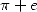unless you add two series who do not have the same form and each converges towards one of the two constants !

I don't know the result for the series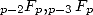... but we can see that we are adding then factorials to the denominator and so we have few chances of finding anything but exotic functions that are impossible to write under simple form! There's already Bessel's function..... Furthermore, they are all close to functions that satisfy similar property to the exponential function.

Notice that in all cases with factorials on the denominator for series of type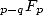with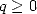, we have a great convergence radius of infinity,, which is nice.... but we already knew it for the exponential function.

If we taje a coefficient to great compare to, the series are less interesting to calculate because they don't converge. For example the serie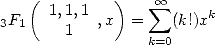(10)

has a convergence radius of zero, that is for any fix number no mater how small it is, the serie won't converge. The factorial is too powerful! It increases to fast compared to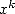... It's also the case generally for the series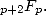This leave us with the intermediate case represented by the function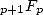who gave us numerous result in the last two century. This is principly due to the fact that the series are homogenuous often to combinations of radicals of polynomials of kind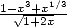or integrals with this kind of things (and in particular the whole class of elliptical integrals). And this is good for us, because we know with this kind of integral we enter completly into the class of integrals which give us some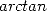and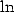, hence! !

The simplest example must be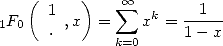(11)

but we can also interest ourself  to the Newton's general binomial formula with rational powers and note that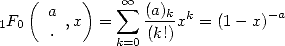(12)

which gives us for example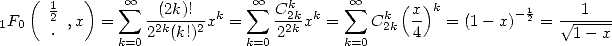(13)

We see here appear some combinationswhose factorials do their bit to be into hypergeometric serie form. The series of typehave a convergence radius between infinity of the seriesand the zero of the series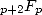i.e. naturally 1 ! We can convince ourself by taking the ratio of the terms of the serie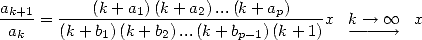(14)

Acording to Alembert's rule, the limit of those ratio is the inverse of the radius of convergence multiplied by, so here this radius is 1.

The derivative of a hypergeometric serie give another hypergeometic serie as the following formula illustrate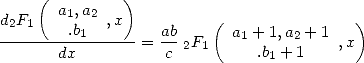(15)

The hypegeometric series are solutions to differential equation of order(using the differential of order), the above example was just a firt illustration. This property sometimes make the calculation of certain hypergeometric series hard, who are equal to very annoying integrals, or even when we don't really know which integral represent it! Those a bit too many functions quickly interested mathematicians who found clever trick to make algorithm to allow indirect calculation of certain hypergeometric series. One of the first method was by Sister Céline , then one by Gosper (yes, yet him again! ! !) and finaly it ended with the algorithm by Wilf-Zeilberger whose method, while not very complicated, is a bit hard and long to explain here...

Many are the hypergeometric function, surely! Imagine that certain very simple and well known series are particular case of them. For example we have seen appear in the formula 12 and 11 powers and combinations. We can also notice that a serie like the one by Leibniz-Gregory is written under a hypergeometric serie form :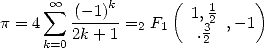(Leibniz-Gregory)

which leave us to think that all series involving only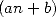terms can also be written under the form of hypergeometric series with the corresponding parameter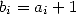(in the above example,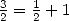.)